Search by Topic

Resources tagged with Multiplication & division similar to Number Lines:

Filter by: Content type:
Age range:
Challenge level:

There are 118 results

Broad Topics > Calculations and Numerical Methods > Multiplication & division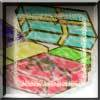Age 7 to 11 Challenge Level:

Use the information to work out how many gifts there are in each pile.The Deca Tree

Age 7 to 11 Challenge Level:

Find out what a Deca Tree is and then work out how many leaves there will be after the woodcutter has cut off a trunk, a branch, a twig and a leaf.What Two ...?

Age 7 to 11 Short Challenge Level:

56 406 is the product of two consecutive numbers. What are these two numbers?Rabbits in the Pen

Age 7 to 11 Challenge Level:

Using the statements, can you work out how many of each type of rabbit there are in these pens?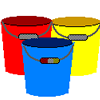Buckets of Thinking

Age 7 to 11 Challenge Level:

There are three buckets each of which holds a maximum of 5 litres. Use the clues to work out how much liquid there is in each bucket.Divide it Out

Age 7 to 11 Challenge Level:

What is the lowest number which always leaves a remainder of 1 when divided by each of the numbers from 2 to 10?Napier's Bones

Age 7 to 11 Challenge Level:

The Scot, John Napier, invented these strips about 400 years ago to help calculate multiplication and division. Can you work out how to use Napier's bones to find the answer to these multiplications?A-magical Number Maze

Age 7 to 11 Challenge Level:

This magic square has operations written in it, to make it into a maze. Start wherever you like, go through every cell and go out a total of 15!Ducking and Dividing

Age 7 to 11 Challenge Level:

Your vessel, the Starship Diophantus, has become damaged in deep space. Can you use your knowledge of times tables and some lightning reflexes to survive?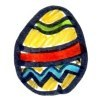How Many Eggs?

Age 7 to 11 Challenge Level:

Peter, Melanie, Amil and Jack received a total of 38 chocolate eggs. Use the information to work out how many eggs each person had.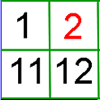What's Left?

Age 5 to 7 Challenge Level:

Use this grid to shade the numbers in the way described. Which numbers do you have left? Do you know what they are called?The Pied Piper of Hamelin

Age 7 to 11 Challenge Level:

This problem is based on the story of the Pied Piper of Hamelin. Investigate the different numbers of people and rats there could have been if you know how many legs there are altogether!Tables Teaser

Age 5 to 7 Challenge Level:

How will you work out which numbers have been used to create this multiplication square?Zargon Glasses

Age 7 to 11 Challenge Level:

Zumf makes spectacles for the residents of the planet Zargon, who have either 3 eyes or 4 eyes. How many lenses will Zumf need to make all the different orders for 9 families?X Is 5 Squares

Age 7 to 11 Challenge Level:

Can you arrange 5 different digits (from 0 - 9) in the cross in the way described?Tom's Number

Age 7 to 11 Challenge Level:

Work out Tom's number from the answers he gives his friend. He will only answer 'yes' or 'no'.Special 24

Age 7 to 11 Challenge Level:

Find another number that is one short of a square number and when you double it and add 1, the result is also a square number.Arranging the Tables

Age 7 to 11 Challenge Level:

There are 44 people coming to a dinner party. There are 15 square tables that seat 4 people. Find a way to seat the 44 people using all 15 tables, with no empty places.Sam's Quick Sum

Age 7 to 11 Challenge Level:

What is the sum of all the three digit whole numbers?The 24 Game

Age 7 to 11 Challenge Level:

There are over sixty different ways of making 24 by adding, subtracting, multiplying and dividing all four numbers 4, 6, 6 and 8 (using each number only once). How many can you find?Surprising Split

Age 7 to 11 Challenge Level:

Does this 'trick' for calculating multiples of 11 always work? Why or why not?The Clockmaker's Birthday Cake

Age 7 to 11 Challenge Level:

The clockmaker's wife cut up his birthday cake to look like a clock face. Can you work out who received each piece?What Is Ziffle?

Age 7 to 11 Challenge Level:

Can you work out what a ziffle is on the planet Zargon?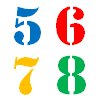How Old?

Age 7 to 11 Challenge Level:

Cherri, Saxon, Mel and Paul are friends. They are all different ages. Can you find out the age of each friend using the information?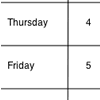Today's Date - 01/06/2009

Age 5 to 11 Challenge Level:

What do you notice about the date 03.06.09? Or 08.01.09? This challenge invites you to investigate some interesting dates yourself.Fingers and Hands

Age 7 to 11 Challenge Level:

How would you count the number of fingers in these pictures?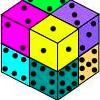Six Numbered Cubes

Age 7 to 11 Challenge Level: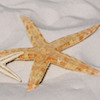Starfish Spotting

Age 5 to 7 Challenge Level:

How many starfish could there be on the beach, and how many children, if I can see 28 arms?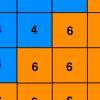Six Ten Total

Age 7 to 11 Challenge Level:

This challenge combines addition, multiplication, perseverance and even proof.Domino Numbers

Age 7 to 11 Challenge Level:

Can you see why 2 by 2 could be 5? Can you predict what 2 by 10 will be?Square Subtraction

Age 7 to 11 Challenge Level:

Look at what happens when you take a number, square it and subtract your answer. What kind of number do you get? Can you prove it?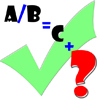Remainders

Age 7 to 11 Challenge Level:

I'm thinking of a number. When my number is divided by 5 the remainder is 4. When my number is divided by 3 the remainder is 2. Can you find my number?Escape from the Castle

Age 7 to 11 Challenge Level:

Skippy and Anna are locked in a room in a large castle. The key to that room, and all the other rooms, is a number. The numbers are locked away in a problem. Can you help them to get out?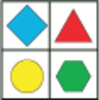Shapes in a Grid

Age 7 to 11 Challenge Level:

Can you find which shapes you need to put into the grid to make the totals at the end of each row and the bottom of each column?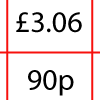How Much Did it Cost?

Age 7 to 11 Challenge Level:

Use your logical-thinking skills to deduce how much Dan's crisps and ice-cream cost altogether.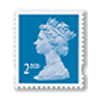Penta Post

Age 7 to 11 Challenge Level:

Here are the prices for 1st and 2nd class mail within the UK. You have an unlimited number of each of these stamps. Which stamps would you need to post a parcel weighing 825g?It Was 2010!

Age 5 to 11 Challenge Level:

If the answer's 2010, what could the question be?Being Resilient - Primary Number

Age 5 to 11 Challenge Level:

Number problems at primary level that may require resilience.Number Tracks

Age 7 to 11 Challenge Level:

Ben’s class were cutting up number tracks. First they cut them into twos and added up the numbers on each piece. What patterns could they see?Odds and Threes

Age 7 to 11 Challenge Level:

A game for 2 people using a pack of cards Turn over 2 cards and try to make an odd number or a multiple of 3.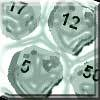Asteroid Blast

Age 7 to 11 Challenge Level:

A game for 2 people. Use your skills of addition, subtraction, multiplication and division to blast the asteroids.The Money Maze

Age 7 to 11 Challenge Level:

Go through the maze, collecting and losing your money as you go. Which route gives you the highest return? And the lowest?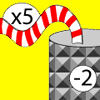Function Machines

Age 7 to 11 Challenge Level:

If the numbers 5, 7 and 4 go into this function machine, what numbers will come out?Countdown

Age 7 to 14 Challenge Level:

Here is a chance to play a version of the classic Countdown Game.Cows and Sheep

Age 7 to 11 Challenge Level:

Use your logical reasoning to work out how many cows and how many sheep there are in each field.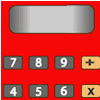Forgot the Numbers

Age 7 to 11 Challenge Level:

On my calculator I divided one whole number by another whole number and got the answer 3.125. If the numbers are both under 50, what are they?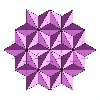Next Number

Age 7 to 11 Short Challenge Level:

Find the next number in this pattern: 3, 7, 19, 55 ...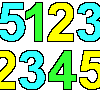Sept03 Sept03 Sept03

Age 7 to 11 Challenge Level:

This number has 903 digits. What is the sum of all 903 digits?Book Codes

Age 7 to 11 Challenge Level:

Look on the back of any modern book and you will find an ISBN code. Take this code and calculate this sum in the way shown. Can you see what the answers always have in common?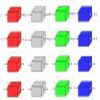Machines

Age 7 to 11 Challenge Level:

What is happening at each box in these machines?